# 9 Mathematical Computations Example using Excel Statistical Function

In addition to formulas, another way to conduct mathematical computations in Excel is through functions. Statistical functions apply a mathematical process to a group of cells in a worksheet.

This chapter gives an overview of some very useful statistical functions like: AVERAGE function, AVERAGEIF function, MEDIAN function, SUMIF function, MIN function, MODE function, STEDV function, LARGE function, SMALL function.

### Average

To calculate the average of a range of cells, use the AVERAGE function.

Worked Example:   Sum if not blank in Excel### Averageif

To average cells based on one criteria, use the AVERAGEIF function. For example, to calculate the average excluding zeros.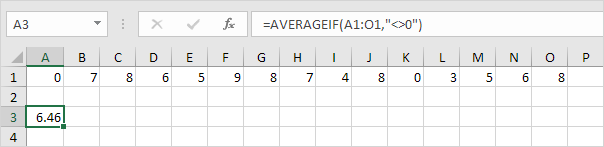Note: <> means not equal to. The AVERAGEIF function is similar to the SUMIF function.

### Median

To find the median (or middle number), use the MEDIAN function.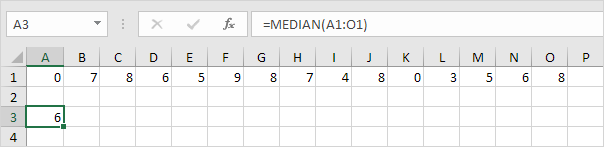Check: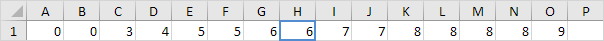### Mode

To find the most frequently occurring number, use the MODE function.

Worked Example:   Sum if greater than in Excel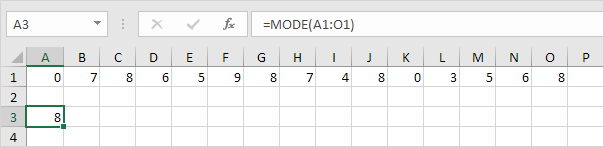### Standard Deviation

To calculate the standard deviation, use the STEDV function.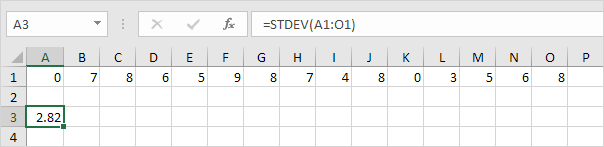### Min

To find the minimum value, use the MIN function.

Worked Example:   Last row number in range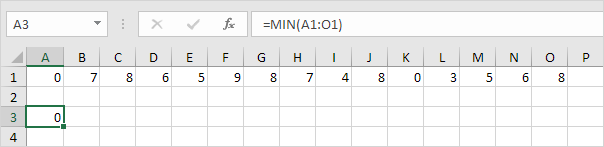### Max

To find the maximum value, use the MAX function.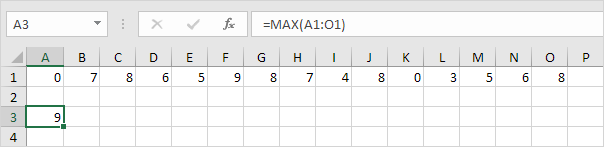### Large

To find the third largest number, use the following LARGE function.Check: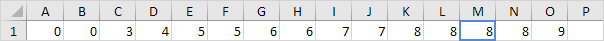### Small

To find the second smallest number, use the following SMALL function.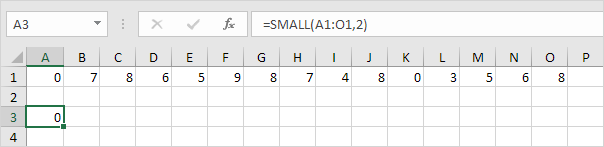Check: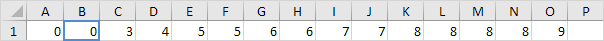Tip: Excel can generate most of these results with the click of a button.